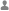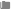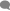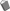## JS知识点：sort()方法的用法，参数以及排序原理Veris分类：Javascript0条评论已被围观 596
sort() 方法用于对数组的元素进行排序,并返回数组。默认排序顺序是根据字符串Unicode码点。

```<script type="text/javascript">

var arr = new Array(6)
arr = "George"
arr = "John"
arr = "Thomas"
arr = "James"
arr = "Martin"

document.write(arr + "<br />")
document.write(arr.sort())

</script>```

```George,John,Thomas,James,Adrew,Martin

```<script type="text/javascript">

var arr = new Array(6)
arr = "10"
arr = "5"
arr = "40"
arr = "25"
arr = "1000"
arr = "1"

document.write(arr + "<br />")
document.write(arr.sort())

</script>```

```10,5,40,25,1000,1
1,10,1000,25,40,5```

```<script type="text/javascript">

function sortNumber(a,b)
{
return a - b
}

var arr = new Array(6)
arr = "10"
arr = "5"
arr = "40"
arr = "25"
arr = "1000"
arr = "1"

document.write(arr + "<br />")
document.write(arr.sort(sortNumber))

</script>```

```10,5,40,25,1000,1
1,5,10,25,40,1000```

```function sortNumber(a,b)
{
return a - b
}
```

```function sortNumber(a,b)
{
return b - a
}
```

sort方法接收一个函数作为参数，这里嵌套一层函数用来接收对象属性名，其他部分代码与正常使用sort方法相同。

```var arr = [
{name:'zopp',age:0},
{name:'gpp',age:18},
{name:'yjj',age:8}
];

function compare(property){
return function(a,b){
var value1 = a[property];
var value2 = b[property];
return value1 - value2;
}
}
console.log(arr.sort(compare('age')))``````sortBy: function(attr,rev){
//第二个参数没有传递 默认升序排列
if(rev ==  undefined){
rev = 1;
}else{
rev = (rev) ? 1 : -1;
}
return function(a,b){
a = a[attr];
b = b[attr];
if(a < b){
return rev * -1;
}
if(a > b){
return rev * 1;
}
return 0;
}
}```

`newArray.sort(sortBy('number',false)) `

V8 引擎 sort 函数只给出了两种排序 InsertionSort 和 QuickSort，数量小于10的数组使用 InsertionSort，比10大的数组则使用 QuickSort。

V8 引擎array源码 710行开始﻿